# Introductory Algebra GlossaryPage 1

#### WATCH ALL SLIDES

Slide 1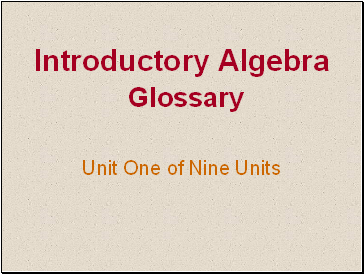Introductory Algebra Glossary

Unit One of Nine Units

Slide 2Introduction

WELCOME

Using the Introductory Algebra Glossary is simple.

Click thru the slide show and check your knowledge of definitions before you display them.

Slide 3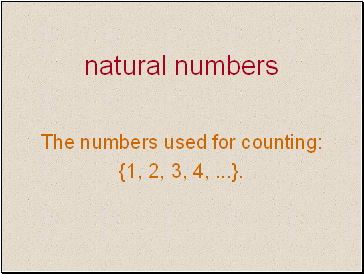## Natural numbers

The numbers used for counting:

{1, 2, 3, 4, .}.

Slide 4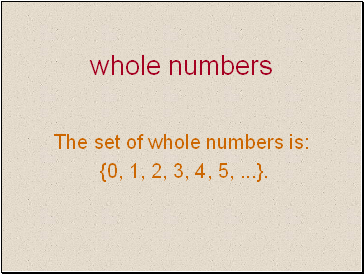## Whole numbers

The set of whole numbers is:

{0, 1, 2, 3, 4, 5, .}.

Slide 5## Numerator

The number above the fraction bar that shows how many equivalent parts are being considered.

Slide 6## Denominator

The number below the fraction bar in a fraction. It shows the number of equal parts in a whole.

Slide 7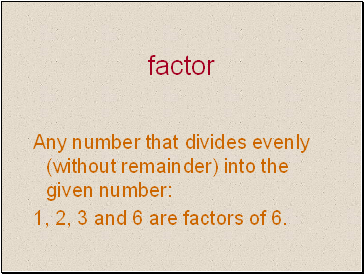## Factor

Any number that divides evenly (without remainder) into the given number:

1, 2, 3 and 6 are factors of 6.

Slide 8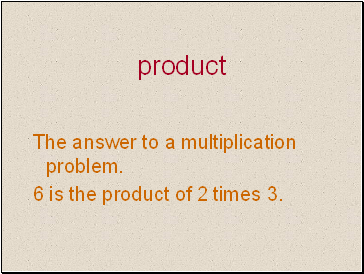## Product

The answer to a multiplication problem.

6 is the product of 2 times 3.

Slide 9## Factored

A number is factored by writing it as the product of two or more numbers.

6 is factored as 2 times 3.

Slide 10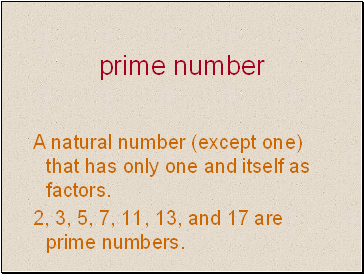## Prime number

A natural number (except one) that has only one and itself as factors.

2, 3, 5, 7, 11, 13, and 17 are prime numbers.

Slide 11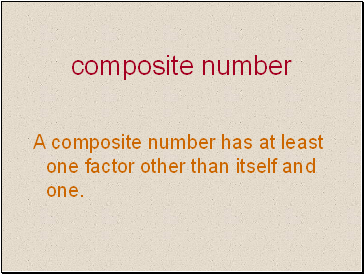## Composite number

A composite number has at least one factor other than itself and one.

Slide 12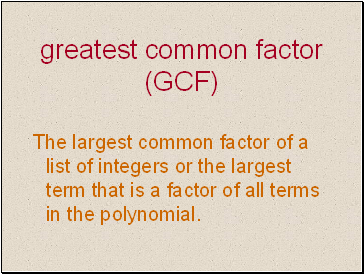## Greatest common factor (GCF)

The largest common factor of a list of integers or the largest term that is a factor of all terms in the polynomial.

Slide 13## Lowest terms

A fraction is in lowest terms when there are no common factors in the numerator and denominator (except 1).

Slide 14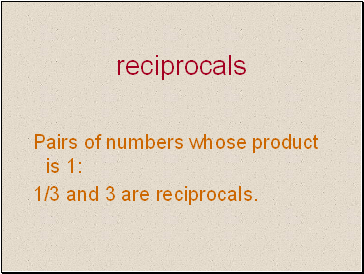Go to page:
1  2  3  4  5